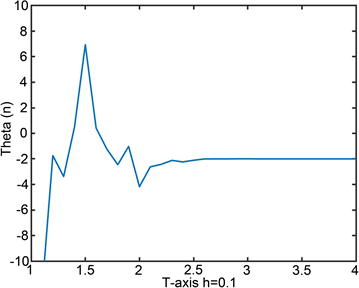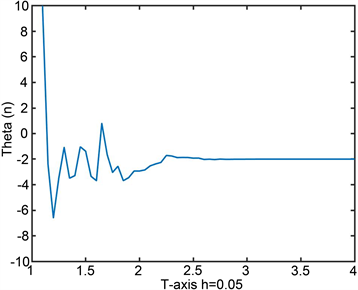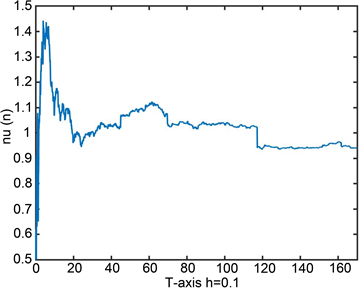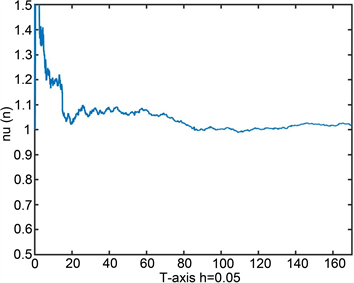﻿ 由α-稳定过程驱动的线性自排斥扩散过程的参数估计

由α-稳定过程驱动的线性自排斥扩散过程的参数估计Parameter Estimation for the Linear Self-Repelling Diffusion Driven by α-Stable Motions

Abstract: In this paper, we consider parameter estimations of the linear self-repelling diffusion, where Mα is a symmetrical α-stable motion (1α＜2). The process is an analogue of the self-repelling diffusion. By using least squares method, we study estimators of unknown parameters, give their asymptotic distributions under the continuous observation and study the accuracy of the estimator.

1. 引言

1992年，Durrett和Rogers  研究了一类增长物模型。在某种条件下，他们建立了如下随机微分方程解的渐近性质：

${X}_{t}={B}_{t}+{\int }_{0}^{t}{\int }_{0}^{s}f\left({X}_{s}-{X}_{u}\right)\text{d}u\text{d}s$

${X}_{t}={X}_{0}+{B}_{t}+{\int }_{0}^{t}\text{d}s{\int }_{ℝ}f\left(-x\right){\mathcal{L}}^{X}\left(s,{X}_{s}+x\right)\text{d}x$

${X}_{t}={B}_{t}-\theta {\int }_{0}^{t}{\int }_{0}^{s}\left({X}_{s}-{X}_{u}\right)\text{d}u\text{d}s+\nu t,\text{ }t\ge 0$

${X}_{t}^{\alpha }={M}_{t}^{\alpha }-\theta {\int }_{0}^{t}{\int }_{0}^{s}\left({X}_{s}^{\alpha }-{X}_{r}^{\alpha }\right)\text{d}r\text{d}s+\nu t$

${Y}_{s}^{\alpha }={\int }_{0}^{t}\left({X}_{s}^{\alpha }-{X}_{r}^{\alpha }\right)\text{d}s,\text{ }t\ge 0$

$\theta$$\nu$ 的最小二乘估计量可以由以下比较函数的最小值求出：

${\rho }_{n}\left(\theta ,\nu \right)={\int }_{0}^{T}{|{X}_{t}{}^{\alpha }-\left(\nu -\theta {Y}_{t}{}^{\alpha }\right)|}^{2}\text{d}t$

${\stackrel{^}{\theta }}_{T}=\frac{{X}_{T}^{\alpha }{\int }_{0}^{T}{Y}_{t}^{\alpha }\text{d}t-T{\int }_{0}^{T}{Y}_{t}^{\alpha }\text{d}{X}_{t}^{\alpha }}{T{\int }_{0}^{T}{\left({Y}_{t}^{\alpha }\right)}^{2}\text{d}t-{\left({\int }_{0}^{T}{Y}_{t}^{\alpha }\text{d}t\right)}^{2}}$

${\stackrel{^}{\nu }}_{T}=\frac{1}{T}\left({X}_{T}^{\alpha }+{\stackrel{^}{\theta }}_{T}{\int }_{0}^{T}{Y}_{t}^{\alpha }\text{d}t\right)$

${\stackrel{^}{\theta }}_{T}\to \theta$

$\stackrel{^}{\nu }\to \nu$

${T}^{1/\alpha -1}{\text{e}}^{-\frac{1}{2}\theta {T}^{2}}\left({\stackrel{^}{\theta }}_{T}-\theta \right)\to -\frac{2{\theta }^{1-1/\alpha }}{{\alpha }^{1/\alpha }}{\stackrel{˜}{M}}_{1}{|{\xi }_{\infty }-\frac{\nu }{\theta }|}^{-1}$ ,

${T}^{1/\alpha -1}\left(\stackrel{^}{\nu }-\nu -\frac{1}{T}{M}_{t}\right)\to \frac{2{\theta }^{-1/\alpha }}{{\alpha }^{1/\alpha }}{\stackrel{˜}{M}}_{1}\mathrm{sgn}\left({\xi }_{\infty }-\frac{\nu }{\theta }\right)$ .

2. 预备知识

$E\left[{\text{e}}^{iu\left({M}_{t}^{\alpha }-{M}_{s}^{\alpha }\right)}\right]={\text{e}}^{-\left(t-s\right)\lambda {|u|}^{\alpha }}$

$\text{d}{X}_{t}^{\alpha }=\text{d}{M}_{t}^{\alpha }-\theta \left({\int }_{0}^{t}\left({X}_{t}^{\alpha }-{X}_{r}^{\alpha }\right)\text{d}r\right)\text{d}t+\nu \text{d}t$ (1)

${h}_{\theta }\left(t,s\right)=\left\{\begin{array}{ll}1-\theta s{\text{e}}^{\frac{1}{2}\theta {s}^{2}}{\int }_{s}^{t}{\text{e}}^{-\frac{1}{2}\theta {u}^{2}}\text{d}u\hfill & t\ge s\hfill \\ 0\hfill & t

${X}_{t}^{\alpha }={\int }_{0}^{t}{h}_{\theta }\left(t,s\right)\text{d}{M}_{s}^{\alpha }+\nu {\int }_{0}^{t}{h}_{\theta }\left(t,s\right)\text{d}s$

3. 强相合性

${\Phi }_{T}:=T{\int }_{0}^{T}{\left({Y}_{t}\right)}^{2}\text{d}t-{\left({\int }_{0}^{T}{Y}_{t}\text{d}t\right)}^{2}$

${\stackrel{^}{\theta }}_{T}=\theta +\frac{1}{{\Phi }_{T}}\left({X}_{T}{\int }_{0}^{T}{Y}_{t}\text{d}t-T{\int }_{0}^{T}{Y}_{t}\text{d}{X}_{t}\right)$ (2)

$\stackrel{^}{\nu }=\nu +\frac{1}{T}{M}_{t}+\left({\stackrel{^}{\theta }}_{T}-\theta \right)\frac{1}{T}{\int }_{0}^{T}{Y}_{t}\text{d}t$ (3)

${Y}_{t}={\int }_{0}^{t}\left({x}_{s}-{x}_{r}\right)\text{d}s={\int }_{0}^{t}s\text{d}{x}_{s}={\int }_{0}^{t}s\text{d}{M}_{s}-\theta {\int }_{0}^{t}s{Y}_{s}\text{d}s+\nu {\int }_{0}^{t}s\text{d}s$

${Y}_{t}={\text{e}}^{-\frac{1}{2}\theta {t}^{2}}{\int }_{0}^{t}s{\text{e}}^{\frac{1}{2}\theta {s}^{2}}\text{d}{M}_{s}+\frac{\nu }{\theta }\left(1-{\text{e}}^{-\frac{1}{2}\theta {t}^{2}}\right)={\text{e}}^{-\frac{1}{2}\theta {t}^{2}}{\xi }_{t}+\frac{\nu }{\theta }\left(1-{\text{e}}^{-\frac{1}{2}\theta {t}^{2}}\right)$ (4)

$T{\text{e}}^{\frac{1}{2}\theta {T}^{2}}{\int }_{0}^{T}{Y}_{t}\text{d}t\to -\frac{1}{\theta }\left({\xi }_{\infty }-\frac{\nu }{\theta }\right)$

$T{\text{e}}^{\frac{1}{2}\theta {T}^{2}}{\int }_{0}^{T}{Y}_{t}\text{d}t=T{\text{e}}^{\frac{1}{2}\theta {T}^{2}}{\int }_{0}^{t}{\text{e}}^{-\frac{1}{2}\theta {t}^{2}}{\xi }_{t}\text{d}t+\frac{\nu }{\theta }{T}^{2}{\text{e}}^{\frac{1}{2}\theta {T}^{2}}-T{\text{e}}^{\frac{1}{2}\theta {T}^{2}}{\int }_{0}^{T}\frac{\nu }{\theta }{\text{e}}^{-\frac{1}{2}\theta {t}^{2}}\text{d}t\to -\frac{1}{\theta }\left({\xi }_{\infty }-\frac{\nu }{\theta }\right)$

${\xi }_{\infty }-{\xi }_{t}={\int }_{t}^{\infty }s{\text{e}}^{\frac{1}{2}\theta {s}^{2}}\text{d}Ms=-t{\text{e}}^{\frac{1}{2}\theta {t}^{2}}{M}_{t}^{2}-{\int }_{0}^{\infty }{M}_{s}^{\alpha }\left(1+\theta {s}^{2}\right){\text{e}}^{\frac{1}{2}\theta {s}^{2}}\text{d}s$

${\text{e}}^{\theta {T}^{2}}{\Phi }_{T}\to -\frac{1}{2\theta }{\left({\xi }_{\infty }-\frac{\nu }{\theta }\right)}^{2}$

${T}^{-2}{\text{e}}^{\frac{1}{2}\theta {T}^{2}}\left({M}_{T}{\int }_{0}^{T}{Y}_{t}\text{d}t-T{\int }_{0}^{T}{Y}_{t}\text{d}{M}_{t}\right)\to 0\text{ }\left(T\to \infty \right)\text{ }a.s.$

${T}^{-2}{\text{e}}^{\frac{1}{2}\theta {T}^{2}}\left(T{Y}_{t}{M}_{t}\right)=\frac{{M}_{t}}{T}\cdot \left({\text{e}}^{\frac{1}{2}\theta {T}^{2}}{Y}_{t}\right)\to 0$

${T}^{-2}{\text{e}}^{\frac{1}{2}\theta {T}^{2}}\left(T{\int }_{0}^{T}t{M}_{t}{\text{e}}^{-\frac{1}{2}\theta {t}^{2}}{\xi }_{t}\text{d}t\right)={T}^{-1}{\text{e}}^{\frac{1}{2}\theta {T}^{2}}{\int }_{0}^{T}t{M}_{t}{\text{e}}^{-\frac{1}{2}\theta {t}^{2}}{\xi }_{t}\text{d}t\to 0$

${T}^{-2}{\text{e}}^{\frac{1}{2}\theta {T}^{2}}\left(T{\int }_{0}^{T}t{\text{e}}^{-\frac{1}{2}\theta {t}^{2}}{M}_{t}\text{d}t\right)={T}^{-1}{\text{e}}^{\frac{1}{2}\theta {T}^{2}}{\int }_{0}^{T}t{\text{e}}^{-\frac{1}{2}\theta {t}^{2}}{M}_{t}\text{d}t\to 0$

${\stackrel{^}{\theta }}_{T}-\theta =\frac{1}{{\text{e}}^{\theta {T}^{2}}{\Phi }_{T}}{\text{e}}^{\theta {T}^{2}}\left({M}_{t}{\int }_{0}^{T}{Y}_{t}\text{d}t-T{\int }_{0}^{t}{Y}_{t}\text{d}{M}_{t}\right)\to 0\text{\hspace{0.17em}}\text{\hspace{0.17em}}\text{\hspace{0.17em}}\text{\hspace{0.17em}}a.s.$

$\begin{array}{c}{\stackrel{^}{\nu }}_{T}-\nu =\frac{1}{T}{M}_{t}+\left({\stackrel{^}{\theta }}_{T}-\theta \right)\frac{1}{T}{\int }_{0}^{T}{Y}_{t}\text{d}t\\ =\frac{1}{T}{M}_{t}+\frac{1}{{\text{e}}^{\theta {T}^{2}}{\Phi }_{T}}{T}^{-2}{\text{e}}^{\frac{1}{2}\theta {T}^{2}}\left({M}_{T}{\int }_{0}^{T}{Y}_{t}\text{d}t-T{\int }_{0}^{T}{Y}_{t}\text{d}{M}_{T}\right)T{\text{e}}^{\frac{1}{2}\theta {T}^{2}}\to 0\text{ }a.s.\end{array}$

4. 渐近分布

${T}^{1/\alpha }{\text{e}}^{\frac{1}{2}\theta {T}^{2}}{\left({\int }_{0}^{T}{|{Y}_{t}|}^{\alpha }\text{d}t\right)}^{1/\alpha }={\left(T{\text{e}}^{\frac{\alpha }{2}\theta {T}^{2}}{\int }_{0}^{T}{|{Y}_{t}|}^{\alpha }\text{d}t\right)}^{1/\alpha }\to -\frac{1}{{\left(\alpha \theta \right)}^{1/\alpha }}|{\xi }_{\infty }-\frac{\nu }{\theta }|$

$\begin{array}{c}{T}^{1/\alpha -1}{\text{e}}^{-\frac{1}{2}\theta {T}^{2}}\left({\stackrel{^}{\theta }}_{T}-\theta \right)=\frac{1}{{\text{e}}^{\theta {T}^{2}}{\Phi }_{T}}\left({T}^{1/\alpha -1}{\text{e}}^{\frac{1}{2}\theta {T}^{2}}{M}_{t}{\int }_{0}^{T}{Y}_{t}\text{d}t-{T}^{1/\alpha }{\text{e}}^{\frac{1}{2}\theta {T}^{2}}{\int }_{0}^{T}{Y}_{t}\text{d}{M}_{t}\right)\\ =\frac{1}{{\text{e}}^{\theta {T}^{2}}{\Phi }_{T}}\left[{Υ}_{1}\left(T\right)-{Υ}_{2}\left(T\right)\right]\end{array}$

${Υ}_{1}\left(T\right)={T}^{1/\alpha -1}{\text{e}}^{\frac{1}{2}\theta {T}^{2}}\frac{{M}_{t}}{T}T{\int }_{0}^{T}{Y}_{t}\text{d}t\to 0\text{ }a.s.$

${Υ}_{2}\left(T\right)={T}^{1/\alpha }{\text{e}}^{\frac{1}{2}\theta {T}^{2}}{\stackrel{˜}{M}}_{{\tau }_{\alpha }\left(T\right)}^{\alpha }=\frac{{\stackrel{˜}{M}}_{{\tau }_{\alpha }\left(T\right)}^{\alpha }}{{\tau }_{\alpha }^{1/\alpha }\left(T\right)}{\tau }_{\alpha }^{1/\alpha }\left(T\right){T}^{1/\alpha }{\text{e}}^{\frac{1}{2}\theta {T}^{2}}$

${Υ}_{2}\left(T\right)~-\frac{1}{{\left(\alpha \theta \right)}^{1/\alpha }}{\stackrel{˜}{M}}_{1}|{\xi }_{\infty }-\frac{\nu }{\theta }|$

${T}^{1/\alpha -1}{\text{e}}^{-\frac{1}{2}\theta {T}^{2}}\left({\stackrel{^}{\theta }}_{T}-\theta \right)\to -\frac{\frac{1}{{\left(\alpha \theta \right)}^{1/\alpha }}{\stackrel{˜}{M}}_{1}|{\xi }_{\infty }-\frac{\nu }{\theta }|}{\frac{1}{2\theta }{\left({\xi }_{\infty }-\frac{\nu }{\theta }\right)}^{2}}=-\frac{2{\theta }^{1-1/\alpha }}{{\alpha }^{1/\alpha }}{\stackrel{˜}{M}}_{1}{|{\xi }_{\infty }-\frac{\nu }{\theta }|}^{-1}$

${T}^{1/\alpha -1}\left(\stackrel{^}{\nu }-\nu -\frac{1}{T}{M}_{t}\right)={T}^{1/\alpha -1}{\text{e}}^{-\frac{1}{2}\theta {T}^{2}}\left(\stackrel{^}{\theta }-\theta \right){\text{e}}^{\frac{1}{2}\theta {T}^{2}}{\int }_{0}^{T}{Y}_{t}\text{d}t\to \frac{2{\theta }^{-1/\alpha }}{{\alpha }^{1/\alpha }}{\stackrel{˜}{M}}_{1}\mathrm{sgn}\left({\xi }_{\infty }-\frac{\nu }{\theta }\right)$

5. 统计模拟

${X}_{t}^{\alpha }={M}_{t}^{\alpha }-\theta {\int }_{0}^{t}{\int }_{0}^{s}\left({X}_{s}^{\alpha }-{X}_{r}^{\alpha }\right)\text{d}r\text{d}s+\nu t$Figure 1. Curve: $\stackrel{^}{\theta }$ when h = 0.01Figure 2. Curve: $\stackrel{^}{\theta }$ when h = 0.05Figure 3. Curve: $\stackrel{^}{\nu }$ when h = 0.1Figure 4. Curve: $\stackrel{^}{\nu }$ when h = 0.1

 Durrett, R. and Rogers, L.C.G. (1992) Asymptotic Behavior of Brownian Polymer. Probability Theory and Related Fields, 92, 337-349.
https://doi.org/10.1007/BF01300560

 Cranston, M. and Le Jan, Y. (1995) Self-Attracting Diffusion: Two Case Studies. Mathematische Annalen, 303, 87-93.
https://doi.org/10.1007/BF01460980

 Benaïm, M., Ciotir, I. and Gauthier, C.-E. (2015) Self-Repelling Diffusions via an Infinite Dimensional Approach. Stochastic Partial Differential Equations: Analysis and Computations, 3, 506-530.
https://doi.org/10.1007/s40072-015-0059-5

 Benaïm, M., Ledoux, M. and Raimond, O. (2002) Self-Interacting Diffusions. Probability Theory and Related Fields, 122, 1-41.
https://doi.org/10.1007/s004400100161

 Cranston, M. and Mountford, T.S. (1996) The Strong Law of Large Numbers for a Brownian Polymer. Annals of Probability, 24, 1300-1323.
https://doi.org/10.1214/aop/1065725183

 Gauthier, C.-E. (2016) Self Attracting Diffusions on a Sphere and Application to a Periodic Case. Electronic Communications in Probability, 21, 1-12.
https://doi.org/10.1214/16-ECP4547

 Herrmann, S. and Roynette, B. (2003) Boundedness and Convergence of Some Self-Attracting Diffusions. Mathematische Annalen, 325, 81-96.
https://doi.org/10.1007/s00208-002-0370-0

 Herrmann, S. and Scheutzow, M. (2004) Rate of Convergence of Some Self-Attracting Diffusions. Stochastic Processes and Their Applications, 111, 41-55.
https://doi.org/10.1016/j.spa.2003.10.012

 Mountford, T. and Tarrès, P. (2008) An Asymptotic Result for Brownian Polymers. Annales de l’IHP Probabilités et Statistiques, 44, 29-46.
https://doi.org/10.1214/07-AIHP113

 Sun, X. and Yan, L. (2020) A Convergence on the Linear Self-Interacting Diffusion Driven by α-Stable Motion, to Appear. Stochastics.
https://doi.org/10.1080/17442508.2020.1869239

 Li, Z. and Ma, C. (2015) Asymptotic Properties of Estimators in a Stable Cox-Ingersoll-Ross Model. Stochastic Processes and Their Applications, 125, 3196-3233.
https://doi.org/10.1016/j.spa.2015.03.002

 Shimizu, Y. (2006) M-Estimation for Discretely Observed Ergodic Diffusion Processes with Infinite Jumps. Statistical Inference for Stochastic Processes, 9, 179-225.
https://doi.org/10.1007/s11203-005-8113-y

 Shimizu, Y. and Yoshida, N. (2006) Estimation of Parameters for Diffusion Processes with Jumps from Discrete Observations. Statistical Inference for Stochastic Processes, 9, 227-277.
https://doi.org/10.1007/s11203-005-8114-x

 Hu, Y. and Long, H. (2009) Least Squares Estimator for Ornstein—Uhlenbeck Processes Driven by α-Stable Motions. Stochastic Processes and their Applications, 119, 2465-2480.
https://doi.org/10.1016/j.spa.2008.12.006

 Applebaum, D. (2004) Lévy Processes and Stochastic Calculus. Cambridge University Press, Cambridge.
https://doi.org/10.1017/CBO9780511755323

 Rosinski, J. and Woyczynski, W.A. (1986) On Itô Stochastic Integration with Respect to P-Stable Motion: Inner Clock, Integrability of Sample Paths, Double and Multiple Integrals. Annals of Probability, 14, 271-286.
https://doi.org/10.1214/aop/1176992627

 Kallenberg, O. (1992) Some Time Change Representations of Stable Integrals, via Predictable Transformations of Local Martingales. Stochastic Processes and Their Applications, 40, 199-223.
https://doi.org/10.1016/0304-4149(92)90012-F

 Protter, P. (2005) Stochastic Integration and Differential Equations. Vol. 21, Springer, Berlin, Heidelberg and New York.
https://doi.org/10.1007/978-3-662-10061-5

Top